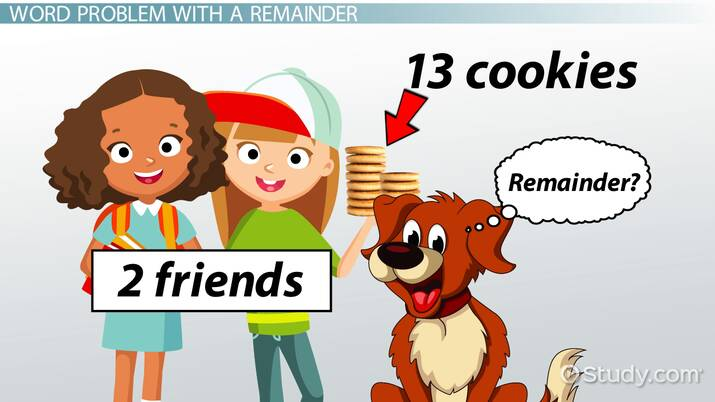# Solving Division Word Problems

Lesson Transcript
Instructor: Francine White

Francine has taught elementary school and is currently an elementary Math specialist. She has two Master's degrees: Curriculum and Instruction in Math K-14 and Educational Leadership

Division word problems...Do these three words make you want to pull your hair out? Never fear! In this lesson, you'll learn four simple steps that will help you solve those dreaded division word problems. Updated: 02/10/2020

## What Is Division?

It's important to start with a simple question and answer: what is division? Quite simply, division means to split something into equal parts. Suppose you had eight cookies to share with a friend evenly. Each of you would get four cookies: 8 / 2 = 4.An error occurred trying to load this video.

Try refreshing the page, or contact customer support.

Coming up next: Solving Division Patterns Over Increasing Place Values

### You're on a roll. Keep up the good work!

Replay
Your next lesson will play in 10 seconds
• 0:04 What Is Division?
• 0:21 Steps to Solving Word Problems
• 0:44 Word Problem with No Remainder
• 1:53 Word Problem With a Remainder
• 3:07 Lesson Summary
Save Save

Want to watch this again later?

Timeline
Autoplay
Autoplay
Speed Speed

## Steps to Solving Word Problems

When you solve a division word problem, you must:

2. Understand what's being divided. This - the number that is being divided - is called the dividend.
3. Understand how the dividend will be divided. In other words, how many equal pieces will the dividend be made into? This number is called the divisor.

Let's get started!

## Word Problem with No Remainder

315 fifth-graders are going on a field trip. If the school purchased 5 buses for the trip, how many students will ride on each bus?

Step 1: To make sure you answer the question, turn the question into a statement: _____ students will ride on each bus.

Step 2: Decide what needs to be divided: 315 students must be divided.

Step 3: Decide how the dividend will be divided: 315 students will be divided among the 5 buses.

The problem is: 315 / 5

Now, let's take a quick minute to review long division. Since we know that there are no groups of 5 in 3, we look at 31. There are 6 groups of 5 in 31. So we put the 6 above the tens place in the dividend.

We then multiply the 6 tens or 60 x 5, to get 300.

When we subtract 300 from 315, the difference is 15. There are three groups of 5 in 15. The 3 goes above the ones place in the dividend.

Step 4: Fill in the blank in your statement: 315 / 5 = 63. 63 students will ride on each bus.

## Word Problem with a Remainder

Sometimes when we divide something, the entire amount does not divide evenly. For example, if you wanted to share 13 cookies with your best friend, each of you would get 6 cookies, but there would be one cookie left over. This is called the remainder.

Let's practice!

To unlock this lesson you must be a Study.com Member.

### Register to view this lesson

Are you a student or a teacher?